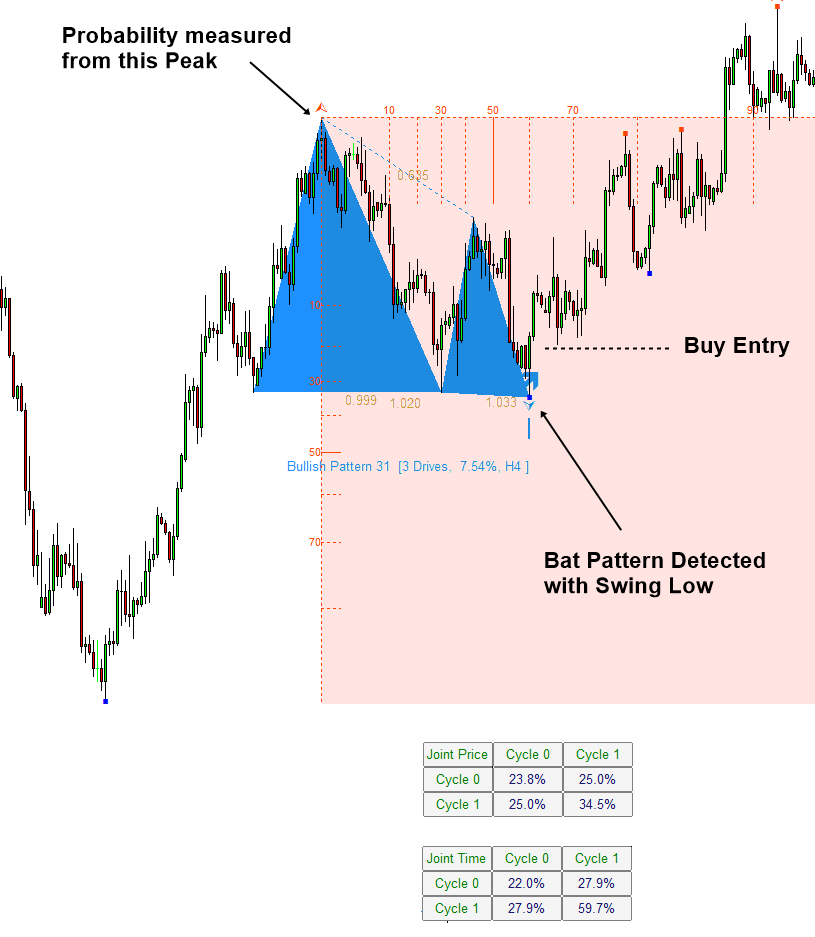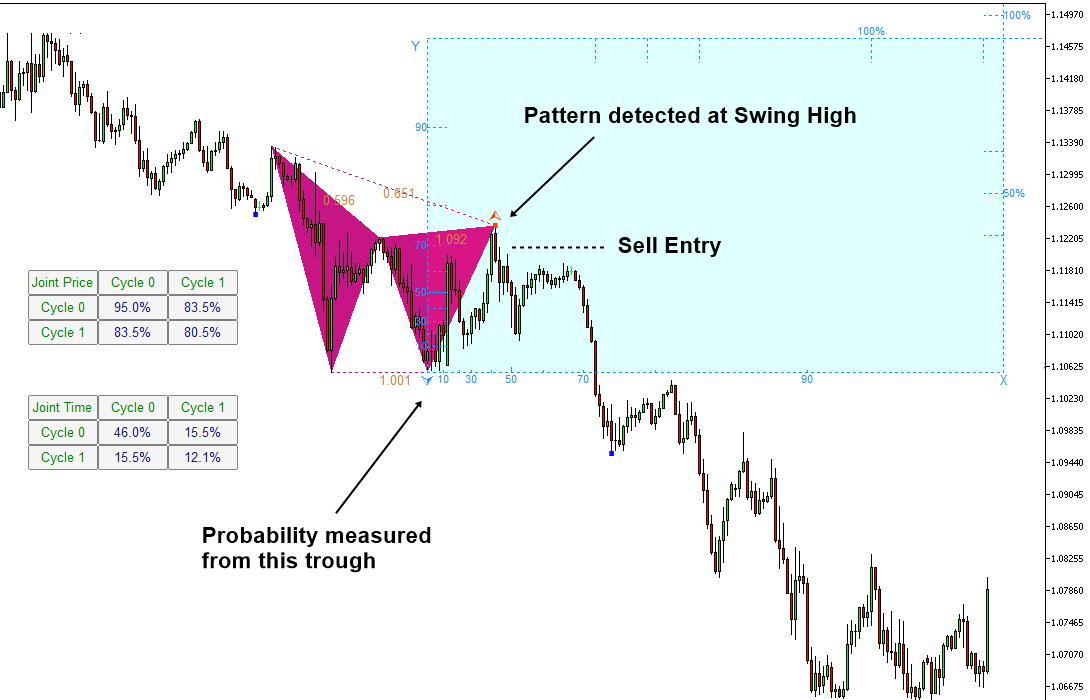# Harmonic Pattern Trading with Turning Point Probability

In this chapter, we will show you how to combine statistical regularity and geometric regularity to trade with Harmonic patterns. In Harmonic Pattern trading, the geometric regularity is recognized with the ratio when several neighbouring fractal triangles are form the certain structure. Once, geometric regularity is recognized by detecting Harmonic Pattern, we detect the statistical regularity with Fibonacci Probability graph to reduce the uncertainty further. Hence, we can improve our accuracy for our trading.

As in the support and resistance, we can consider continuation pattern and reversal pattern for harmonic pattern trading. In continuation pattern, we try to find the harmonic pattern giving us the trading entry in the current trend direction. As you might expect, in the case of continuation pattern, the counter trend movement (i.e. correction) is weak. This will result in the small probability in the joint probability matrix. Hence, we have less conservative criteria in choosing our entry. For example, we will consider the probability 50% as the minimum cut for the continuation pattern.Figure 4.5-1: Buy example of continuation pattern with turning point probability on EURUSD H4

For harmonic pattern trading, we need to have some threshold price level too to trigger our entry. We have more choice on the threshold price level than the case of support and resistance. For example, in the book “Guide to Precision Harmonic Pattern Trading”, we have describe several different approach trading with harmonic patterns. If you have read the part of the book explaining the Pattern Completion Interval and Potential Continuation Zone, then it is fine to use them. At the same time, it is fine to apply the same criteria as in the support and resistance as long as you understand following two points. Firstly, we need the good spacing between the trigger level and the final point D.  Secondly, we need the good Reward to Risk ratio for our trading. As long as you understand these two points, you can choose the most sensible method among them. In this book, we provide the examples using one of these three choices.

• Choice 1: you can use a sensible peak or trough around the support and resistance line.
• Choice 2: you can use the sensible Fibonacci retracement in the left axis of Fibonacci probability graph.
• Choice 3: you can use the probability in the right axis of Fibonacci probability graph when either first or second choice is not available.Figure 4.5-2: Sell example of continuation pattern with turning point probability on EURUSD H1

With Harmonic Pattern, it is possible to trade with reversal pattern. In reversal pattern, we are trading against current trend. Reversal pattern is particularly helpful in picking up the global turning point, where Reward is much higher than Risk. At the same time, we require more skills for trading with reversal pattern. Especially, we recommend applying conservative criterial in our statistical regularity. Over 90% probability is preferred in reversal pattern trading. For example in Figure 4.5-3, we have confirmed AB=CD pattern with 91.1% probability in Large Cycle in time dimension. Joint probability is also high at 83.4%. With higher probability, we can ensure the safety of our trading in the case of reversal pattern.Figure 4.5-3: Buy example of reversal pattern with turning point probability on GBPUSD H1

In Figure 4.5-4, we have also high probability of 91.1% in time dimension. Although it was for small cycle, the probability in price dimension is also high at 88.5%. Confirmation of high probability in time and price dimension provides us more safety in our trading.Figure 4.5-4: Sell example of reversal pattern with turning point probability on GBPUSD D1

Below is the landing page for Fractal Pattern Scanner for MetaTrader 4 and MetaTrader 5. The same products are available on www.mql5.com too.

https://www.mql5.com/en/market/product/49170

https://www.mql5.com/en/market/product/49169